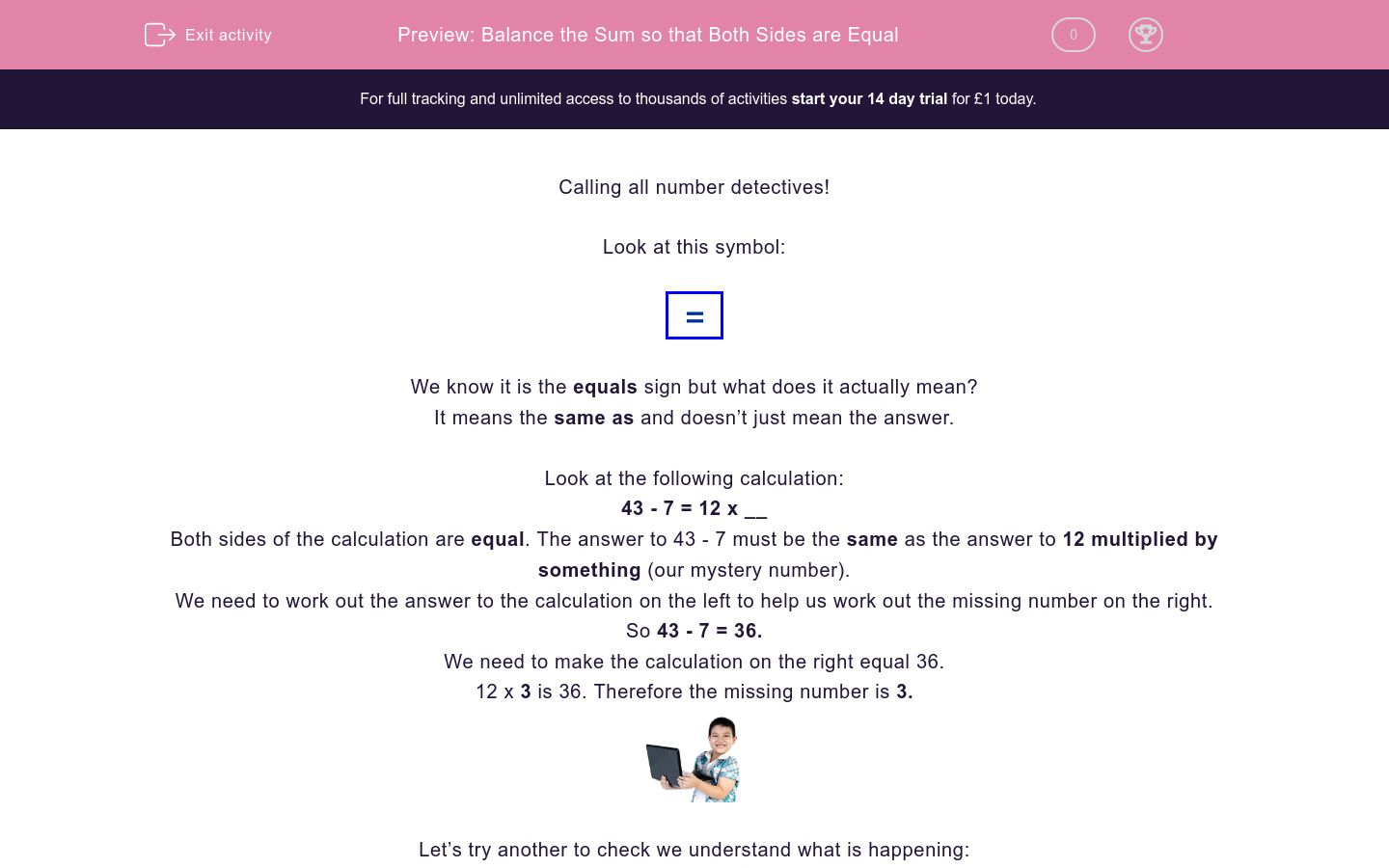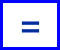# Balance the Sum so that Both Sides are Equal

In this worksheet, students will complete a calculation by adding in the missing number so that both sides are equal. It will strengthen their number skills.Key stage:  KS 2

Curriculum topic:  Verbal Reasoning

Curriculum subtopic:  Complete the Calculation

Difficulty level:### QUESTION 1 of 10

Calling all number detectives!

Look at this symbol:We know it is the equals sign but what does it actually mean?

It means the same as and doesn’t just mean the answer.

Look at the following calculation:

43 - 7 = 12 x __

Both sides of the calculation are equal. The answer to 43 - 7 must be the same as the answer to 12 multiplied by something (our mystery number).

We need to work out the answer to the calculation on the left to help us work out the missing number on the right.

So 43 - 7 = 36.

We need to make the calculation on the right equal 36.

12 x 3 is 36. Therefore the missing number is 3.Let’s try another to check we understand what is happening:

3 x __ = 5 + 7

Here we must work out the right side of the calculation to help us work out the missing number on the left.

So 5 + 7 = 12.

3 x ? = 12

The missing number is 4 as 3 x 4 = 12.

3 x 4 = 5 + 7

Pssstt!! Here’s a handy hint to help you reach superstar status:

Remember that equals means the same as and the answers on either side of the equals sign must be the same.

In this activity, you will be completing sums by finding the missing number.

Good luck!

Are you ready for another challenge, number detective?

Below is a calculation where both sides should be equal.

However, one of the numbers has been taken away by the cheeky number burglar! Can you work out what it is

60 ÷ 5 =  95 - __OK, here's another exciting question to get your teeth into!

Below is a calculation where both sides should be equal.

Can you find the missing number?

122 - 61 = 32 + __Below is another calculation where both sides should be equal.

Can you work out what number has been missed out, number detective?

78 - 46 = 147 - __

And again ...below is a calculation where both sides should be equal.

Can you work out what number has been missed out?

10 x 11 = 71 + __

You're at the halfway point already, number detective!

Below is a calculation where both sides should be equal.

Can you find the missing number?

35 ÷ 5 = 91 - __You're doing brilliantly - don't give up, number detective!

Below is a calculation where both sides should be equal.

Can you work out what the missing number is?

138 - 72 = 11 x __

Here we go again, number detective!

Below is a calculation where both sides should be equal.

Do you know what the missing number is?

64 + 56 = 10 x __You're so near the end of this activity, number detective!

Below is another calculation where both sides should be equal.

Can you work out what the missing number is?

167 - 43 = 52 + __You're on to the last two questions, number detective!

Below is a calculation where both sides should be equal.

Can you use those detecting skills to find out the missing number?

9 x 4 = 3 x __

You've reached the final question - well done, number detective!

Below is a calculation where both sides should be equal.

Can you work out what the mystery number is?

111 + 33 = 51 + __• Question 1

Are you ready for another challenge, number detective?

Below is a calculation where both sides should be equal.

However, one of the numbers has been taken away by the cheeky number burglar! Can you work out what it is

60 ÷ 5 =  95 - __CORRECT ANSWER
83
EDDIE SAYS
How did you find this first one? Did you remember to do it in several stages? First, you need to work out 60 ÷ 5 = 12. Then, you must put the number 12 as the answer on the right-hand side: 95 - __ = 12. Finally, you can find the answer by doing 95 - 12, which makes 83. Ready for some more fun, number-finding questions?
• Question 2

OK, here's another exciting question to get your teeth into!

Below is a calculation where both sides should be equal.

Can you find the missing number?

122 - 61 = 32 + __CORRECT ANSWER
29
EDDIE SAYS
Did you get this one? First, solve the left-hand side: 122 - 61 = 61. Then, put 61 as the answer to the right-hand side: 32 + __ = 61. Finally, to solve this sum you need to do 61 - 32, which gives you 29. Great!
• Question 3

Below is another calculation where both sides should be equal.

Can you work out what number has been missed out, number detective?

78 - 46 = 147 - __

CORRECT ANSWER
115
EDDIE SAYS
Did you work this one out, detective? First of all, do the sum 78 - 46 = 32. Next, do the right-hand side: 147 - __ = 32. This can be worked out by doing 147 - 32, which gives us 115.
• Question 4

And again ...below is a calculation where both sides should be equal.

Can you work out what number has been missed out?

10 x 11 = 71 + __

CORRECT ANSWER
39
EDDIE SAYS
Are you getting the hang of these? First, do the left hand side: 10 x 11 = 110. Now, put 110 as the answer to the right-hand side: 71 + __ = 110. This can be solved by doing 110 - 71, which makes 39. Great work!
• Question 5

You're at the halfway point already, number detective!

Below is a calculation where both sides should be equal.

Can you find the missing number?

35 ÷ 5 = 91 - __CORRECT ANSWER
84
EDDIE SAYS
You're halfway through this activity! To work out the answer here, you do 35 ÷ 5 = 7. Then, put 7 as the answer to the right-hand side: 91 - __ = 7, which can be worked out by doing 91 - 7. Did you get that one OK, number detective?
• Question 6

You're doing brilliantly - don't give up, number detective!

Below is a calculation where both sides should be equal.

Can you work out what the missing number is?

138 - 72 = 11 x __

CORRECT ANSWER
6
EDDIE SAYS
You're collecting lots of missing numbers! For this question, you first have to do 138 - 72 = 66. Then, put 66 as the answer to the right-hand side, and solve the sum 11 x _ = 66. To do this we need to work out how many elevens there are in 66, which is 6!
• Question 7

Here we go again, number detective!

Below is a calculation where both sides should be equal.

Do you know what the missing number is?

64 + 56 = 10 x __CORRECT ANSWER
12
EDDIE SAYS
Let's go through this one carefully. First, find the answer to 64 + 56 = 120. Then, put 120 as the answer to the right-hand side: 10 x _ = 120. To find out the answer, all you now need to do is work out how many tens there are in 120. Excellent!
• Question 8

You're so near the end of this activity, number detective!

Below is another calculation where both sides should be equal.

Can you work out what the missing number is?

167 - 43 = 52 + __CORRECT ANSWER
72
EDDIE SAYS
Nice work detective! Did you break it down into two stages? Firstly, do 167 - 43 = 124. Then, do the right-hand side: 52 + __ = 124, which can be solved by doing 124 - 52. The answer is 72!
• Question 9

You're on to the last two questions, number detective!

Below is a calculation where both sides should be equal.

Can you use those detecting skills to find out the missing number?

9 x 4 = 3 x __

CORRECT ANSWER
12
EDDIE SAYS
How did you do with this one? The left-hand side was testing your four times table: 9 x 4 = 36. Then, all you had to do was put 36 as the answer on the right-hand side and finally, work out how many threes there are in 36 by doing 36 ÷ 3. Let's take a look at the last question!
• Question 10

You've reached the final question - well done, number detective!

Below is a calculation where both sides should be equal.

Can you work out what the mystery number is?

111 + 33 = 51 + __CORRECT ANSWER
93
EDDIE SAYS
OK, let's work through the stages. First, do the left hand side: 111 + 33 = 144. Then, add 144 to the right hand side: 51 + __ = 144. This can be worked out by doing 144 - 51, which is 93. Your mission is complete - for now! Hopefully, you have found that these got easier with practice.
---- OR ----

Sign up for a £1 trial so you can track and measure your child's progress on this activity.

### What is EdPlace?

We're your National Curriculum aligned online education content provider helping each child succeed in English, maths and science from year 1 to GCSE. With an EdPlace account you’ll be able to track and measure progress, helping each child achieve their best. We build confidence and attainment by personalising each child’s learning at a level that suits them.

Start your £1 trial